## Find out force on each member - truss, Mechanical Engineering

Assignment Help:

Find out force on each member - Truss:

A truss is as shown in the figure given below. Find out force on each member and the nature of it.

Sol.: First we calculate support reaction, Draw FBD as shown in figure given below

Determine forces in all members of truss loaded and supported as shown in the figure given below.

Sol.: The reaction at the supports is determined by considering equilibrium of entire truss. As both the external loads are vertical, only vertical component of reaction at hinged ends A required to be considered. As the ?AEC is a right angle ?, having angle AEC = 90º. Then,

AE = AC cos 60º = 5 X 0.5     = 2.5m

CE = AC sin60º = 5 X 0.866 = 4.33m

As? ABE is an equilateral triangle and thus,

AB = BC = AE = 2.5m

The distance of line of action of force 10KN from the joint A,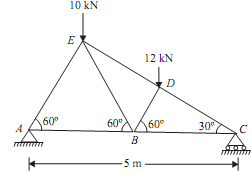AF = AE cos 60º = 2.5 X 0.5 = 1.25m

Again, ?BDC is a right angle ? having angle BDC = 90º. Also,    BC = AC - AB = 5 - 2.5 = 2.5m

BD = BC cos 60º = 2.5 X 0.5 = 1.25m

The distance of line of action of force 12KN from the joint A,

AG = AB + BG = AB + BD cos 60º = 2.5 + 1.25 X 0.5 = 3.125m

By taking moment about end A,

RC  X 5 = 12 X 3.125 + 10 X 1.25 = 50

RC = 10KN                                                                                                                         ...(i)

V = 0, RC  + RA  = 10 + 12 = 22KN

RA = 12KN                                                                                                                       ...(ii)

Joint A:

Consider free body diagram of joint A as shown in figure given below Let, TAE = Force in the member AETAB = Force in member AB Direction of both the forces (TAE and TAB) is taken away from the point A. As the three forces are acting at the joint A. Thus apply lami's theorem at A.TAE/sin 270º = TAB/sin 30º = RA/sin 60º TAE/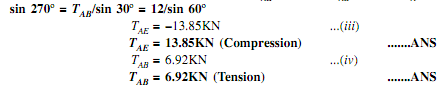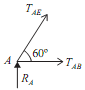Consider free body diagram of joint C as shown in given figure  Assume,
TBC  = Force in member TBC and TCD  = Force in member CD Direction of both the forces (TBC  & TCD) is taken away from point C. As the three forces are acting at joint C. So apply lami's theorem at point C.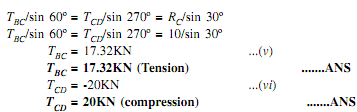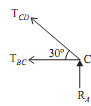=

Joint B:

Consider free body of joint B as shown in fig.

As,                           TAB = 6.92KN

TBC = 17.32KN

Let, TBD  = Force in member BD

TEB  = Force in member EB

Direction of both forces (TBD  and TEB) is taken away from point B. As the four forces are acting at joint B. Thus apply resolution of forces at the B.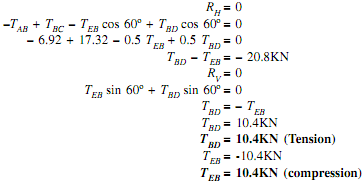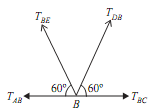Joint D:

Consider free body diagram of joint D as shown in the figure.

As,                         TCD = -20KN

Let,                        TED = Force in member ED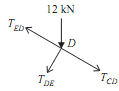Direction of force (TED) is taken away from the point D. As the four forces are acting at joint D. Thus apply resolution of forces at the joint D

Resolve all the forces along EDC,

TED  + 12cos 60º + TCD = 0

TED  + 6 - 20 = 0

TED = 14KN                                                                                                        ...(xi)

TED = 14KN (Tension)                                              .......ANS

 Member AE AB BC CD BD BE DE Force in KN 13.85 6.92 17.32 20 10.4 10.4 14 Nature C = Compression T = Tension C T T C T C T

#### Design the helical compression spring, A helical compression spring prepare...

A helical compression spring prepared of oil tempered carbon steel, is subjected to a load which goes from 600 N to 1600 N. The spring index is 6 and the design factor of safety is

#### Kinematics of rigid bodies, understanding the scope.

understanding the scope.

#### Alumina-silica composite, Alumina-Silica Composite  This composite is a...

Alumina-Silica Composite  This composite is an especially usual refractory material. Due to easy availability and low cost aluminia-silica composite is extensively utilized in

#### Stress relaxation is creep related phenomenon, Stress relaxation is creep r...

Stress relaxation is creep related phenomenon: Problem: What is stress relaxation? Give example. Solution Stress relaxation is creep related phenomenon. It is los

#### Two possible materials for casing, Two Possible Materials for Casing T...

Two Possible Materials for Casing Two possible materials for the casing would be a. Polypropylene or b. An aluminium 1/3 per cent silicon-casting alloy (LM6). Conclu

#### Strength of Materials, AShear Force and Bending Moment Diagrams

AShear Force and Bending Moment Diagrams

#### Explain the zeroth law of thermodynamics, (a) Describe and explain the Zero...

(a) Describe and explain the Zeroth law of thermodynamics. Why it is so called ? What is understand by fixed points of a thermometric scale ? (b) In what respects are the work a

assignment

#### Determine the maximum bending moment, Design the bending moment and shear f...

Design the bending moment and shear force diagram for a simply supported beam of length 9 m and carrying a Uniformly distributed load of 10 KN /m for a distance of 6 m from the lef